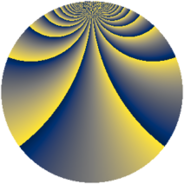# Properties

 Label 1110.2.byLevel $1110$ Weight $2$ Character orbit 1110.by Rep. character $\chi_{1110}(59,\cdot)$ Character field $\Q(\zeta_{36})$ Dimension $912$ Sturm bound $456$

# Related objects

## Defining parameters

 Level: $$N$$ $$=$$ $$1110 = 2 \cdot 3 \cdot 5 \cdot 37$$ Weight: $$k$$ $$=$$ $$2$$ Character orbit: $$[\chi]$$ $$=$$ 1110.by (of order $$36$$ and degree $$12$$) Character conductor: $$\operatorname{cond}(\chi)$$ $$=$$ $$555$$ Character field: $$\Q(\zeta_{36})$$ Sturm bound: $$456$$

## Dimensions

The following table gives the dimensions of various subspaces of $$M_{2}(1110, [\chi])$$.

Total New Old
Modular forms 2832 912 1920
Cusp forms 2640 912 1728
Eisenstein series 192 0 192

## Trace form

 $$912q + 24q^{9} + O(q^{10})$$ $$912q + 24q^{9} - 12q^{15} + 264q^{31} + 24q^{34} - 48q^{40} + 48q^{45} + 96q^{46} - 48q^{49} + 144q^{61} + 96q^{69} + 24q^{70} + 312q^{75} - 216q^{81} - 48q^{91} - 144q^{94} - 240q^{99} + O(q^{100})$$

## Decomposition of $$S_{2}^{\mathrm{new}}(1110, [\chi])$$ into newform subspaces

The newforms in this space have not yet been added to the LMFDB.

## Decomposition of $$S_{2}^{\mathrm{old}}(1110, [\chi])$$ into lower level spaces

$$S_{2}^{\mathrm{old}}(1110, [\chi]) \cong$$ $$S_{2}^{\mathrm{new}}(555, [\chi])$$$$^{\oplus 2}$$